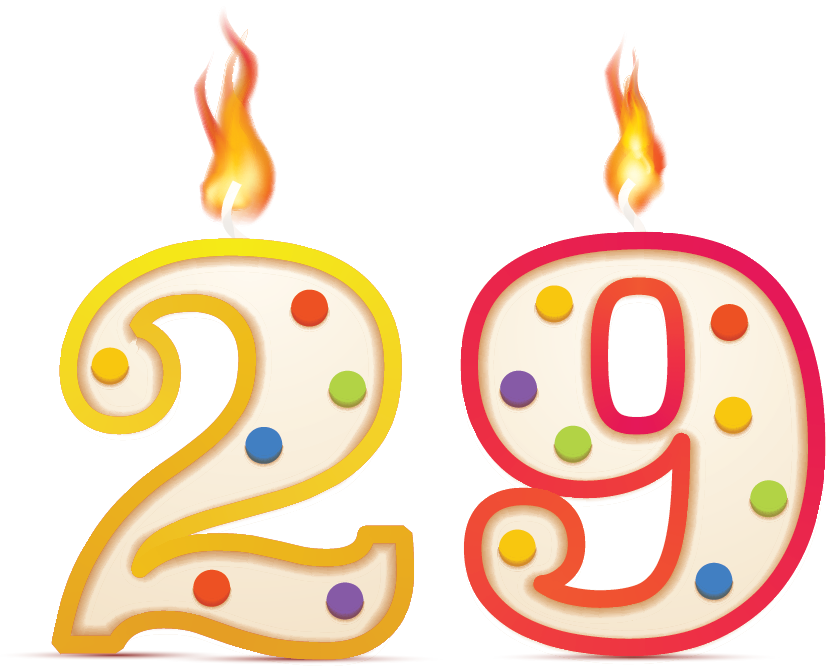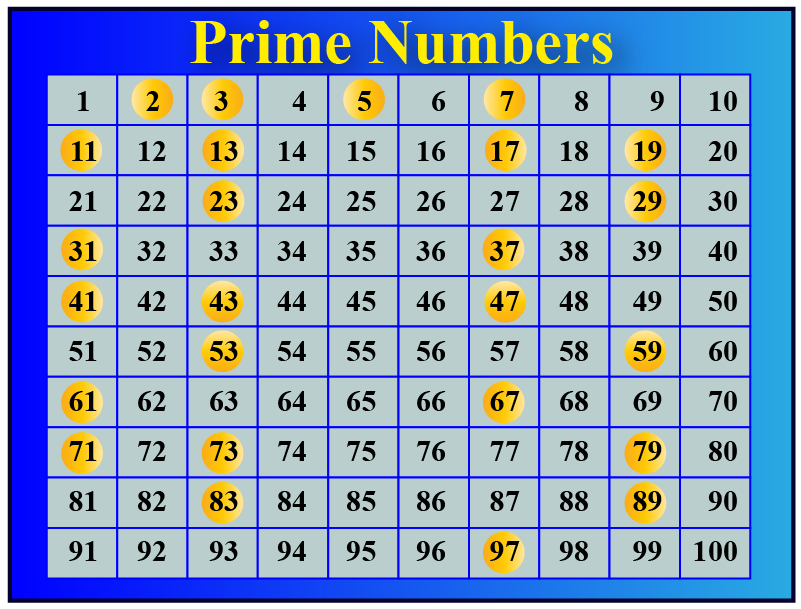# Is 29 A Prime Number

Is 29 A Prime Number
Go back to  'Prime Numbers'

Prime numbers have only 2 factors 1 and the number itself. Do you know if 29 is a prime number? What about other numbers like 19, 49,  39 ? is 39 a prime? is 19 a prime? is 49 a prime? Check out the interactive questions and solved examples to know more about the lesson and try your hand at solving a few interesting practice questions at the end of the page.## Is 29 a Prime Number?

The number which is only divisible by either $$1$$ or by itself is a prime number.

or In other words "a number that is a multiple of only 1 and itself.

For example: Is 29 prime?

The answer is yes. Because it only has two factors as $$1$$ and $$29$$

Let us take one more example: Is $$19$$ a prime number?

The answer will be yes, as it only has $$1$$ and only $$19$$ as the number that can completely divide it leaving no remainder behind.

$$39$$ is a prime number.

Do you know why?

$$39$$ is completely divisible by $$1$$ and $$39$$ only.

This means it exactly has two factors which makes it a prime number.

Let us take an example of another number 49

Is 49 a prime number?

1 and 49 are factors of 49

But, Wait!  $$7 \times 7 is 49$$ which means 7 is also a factor of 49. So, 49 has 3 factors 1,7, and 49. So it is not a prime number. It is a composite number.

In the Number chart below, few numbers are circled in yellow.

Can you verify if all these numbers are prime numbers?## Is 29 a Composite Number?

Composite numbers have factors other than 1 and the number itself. other factors

29 is a prime number. we can't get 29 as a product multiplying two whole numbers other than 1 and 29Tips and Tricks
• 2 is the only even prime number.
• Any two-digit number that ends with 0 or 5 is NOT a prime number because these numbers will be divisible by 5
• If the sum of the digits of a number (other than 3) is divisible by 3 then the number is NOT a prime number.

## Solved Examples

 Example 1

Susan was finishing her school work with her mom, but she had a doubt that out of $$4$$, $$7$$ and $$10$$ which one is a prime number?Solution

Her mother told her to write all the factors of the numbers given.

susan started writing $$4$$ = $$1$$, $$2$$, $$4$$

$$7$$ = $$1$$, $$7$$

$$10$$ = $$1$$,$$2$$,$$5$$,$$10$$

Her mother then told: Susan now have a look at all the numbers and point the number having only two factors

Susan clearly understood that out of all the three numbers $$7$$ is a prime number.

 $$\therefore$$ $$7$$ is a prime number
 Example 2

Help benny solve:

Is 31 prime or NOT?Solution

The given number is 31

Can you find two numbers such that their product is 31?

only $$1 \times 31$$ gives a product 31

Thus 31 has exactly two factors.

Since the number of factors of 31 is exactly 2, it is a prime number.

 $$\therefore$$ $$31$$ is a prime number

## Interactive Questions

Here are a few activities for you to practice.Challenging Question
•  Tim has 49 flowers and Kate has 31 flowers. Who can arrange the flowers in rows such that each row has the same number of flowers?

## Let's Summarize

The mini-lesson is targeted at the fascinating concept of prime numbers. The math journey around prime numbers starts with what a student already knows, and goes on to creatively crafting a fresh concept in the young minds. Done in a way that not only it is relatable and easy to grasp, but also will stay with them forever. Here lies the magic with Cuemath.

At Cuemath, our team of math experts is dedicated to making learning fun for our favorite readers, the students!

Through an interactive and engaging learning-teaching-learning approach, the teachers explore all angles of a topic.

Be it worksheets, online classes, doubt sessions, or any other form of relation, it’s the logical thinking and smart learning approach that we at Cuemath believe in.

## 1. What is a coprime number

Numbers that have only 1 as their common factor  are called coprime numbers

For example, $$18$$ and $$35$$ are coprime numbers as they only share $$1$$ as a common factor.

## 2. Why is 29 a prime number?

29 is a prime number as it only has two factors as 1 and 29

## 3. Is 7 prime yes or no?

7 is a prime number. It only has two factors: 7 and 1

More Important Topics
Numbers
Algebra
Geometry
Measurement
Money
Data
Trigonometry
Calculus